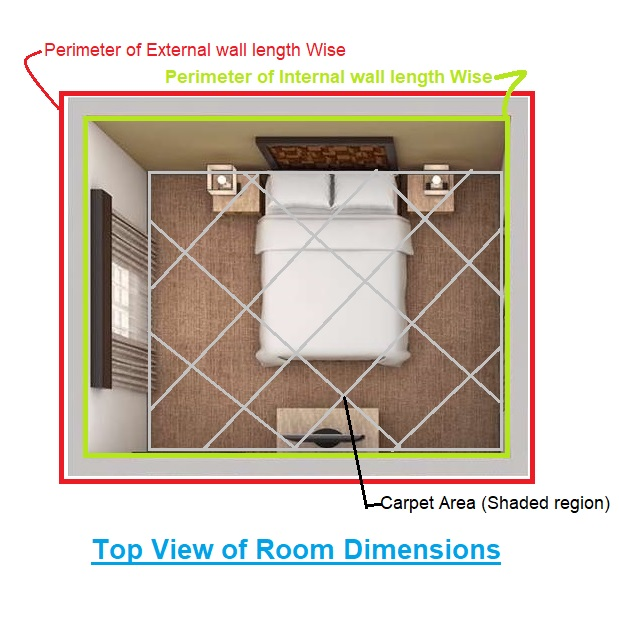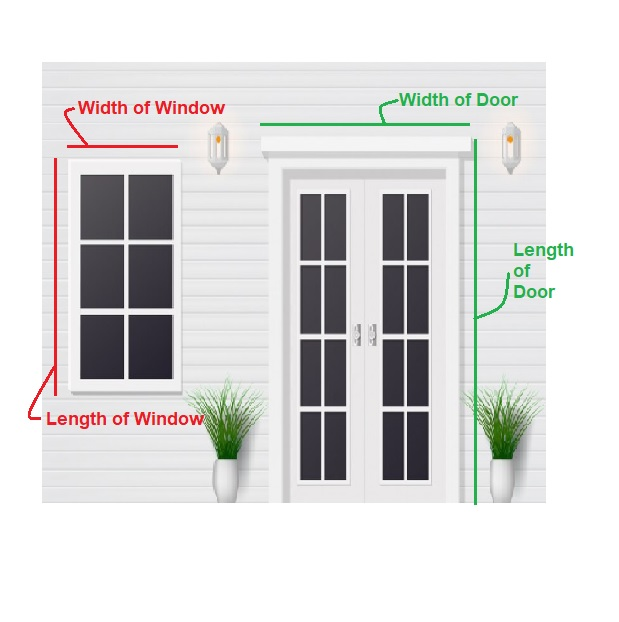Work Hours
Monday to Friday: 7AM - 7PM
Weekend: 10AM - 5PM

# Paint Quantity Calculator

## Calculations for Paint Quantity Calculator

Let’s understand with the help of an example, how to use the above Paint quantity calculator.Assumptions For Example

• Perimeter of Internal wall length Wise = 20 m = l
• Perimeter of External wall length Wise = 30 m = b
• Height of wall = 5 m = h
• Carpet Area = 20 m2 = A
• Window (Length, Width, Quantity) = (2 m , 2 m , 1)
• Door (Length, Width, Quantity) = (3 m , 2 m , 1)
• Putty consumption for 2 coat = 1.3 m2/Kg
• Primer consumption for 1 coat = 9 m2/L
• Paint consumption for 2 coat = 6.5 m2/L

Calculation Procedure

• Step 1: Calculation of Surface Area to be Painted
Surface Area=(((l +b) x h)+A -(Area of window +Area of Door))
= ((20+30)x5 + 20 – (2x2x1) – (3x2x1)) = 230 m2

• Step 2: Calculation of Amount of Putty
Amount of Putty for 2 coat = Surface Area / 1.3
= 230 / 1.3
= 177 Kg

• Step 3: Calculation of Amount of Primer
Amount of Primer for 1 coat = Surface Area / 9
= 230 / 9
= 25.55 L

• Step 4: Calculation of Amount of Paint
Amount of Paint for 2 coat = Surface Area / 6.5
= 230 / 6.5
= 36 L

Final Result

Surface Area to be Painted = 230 m2
Amount of Putty = 177 Kg
Amount of Primer = 25.55 L
Amount of Paint = 36 L

Try Onsite, #1 Tool For Site Tracking

/** zohoform script */